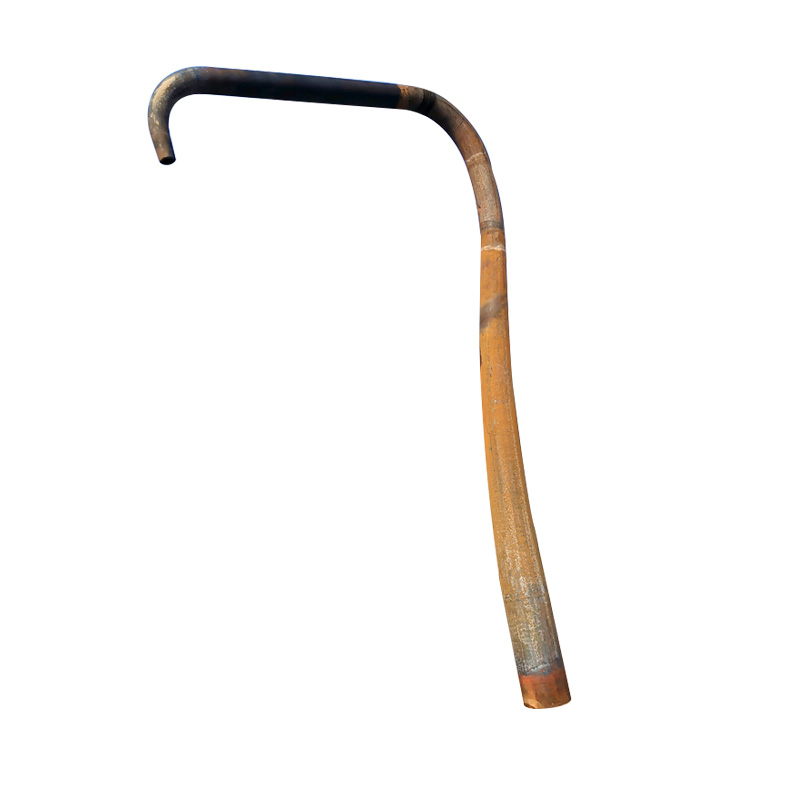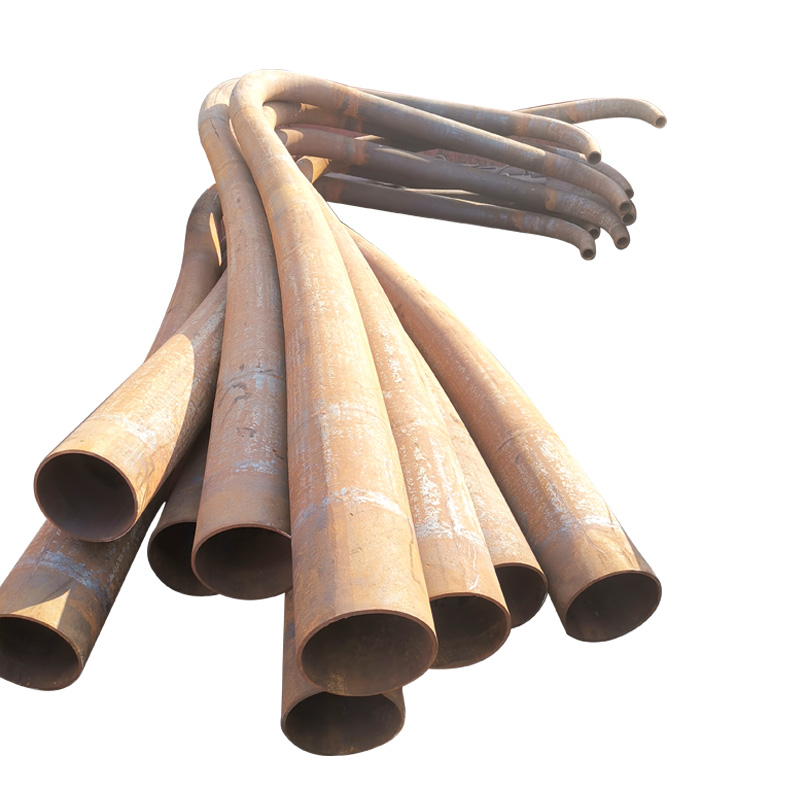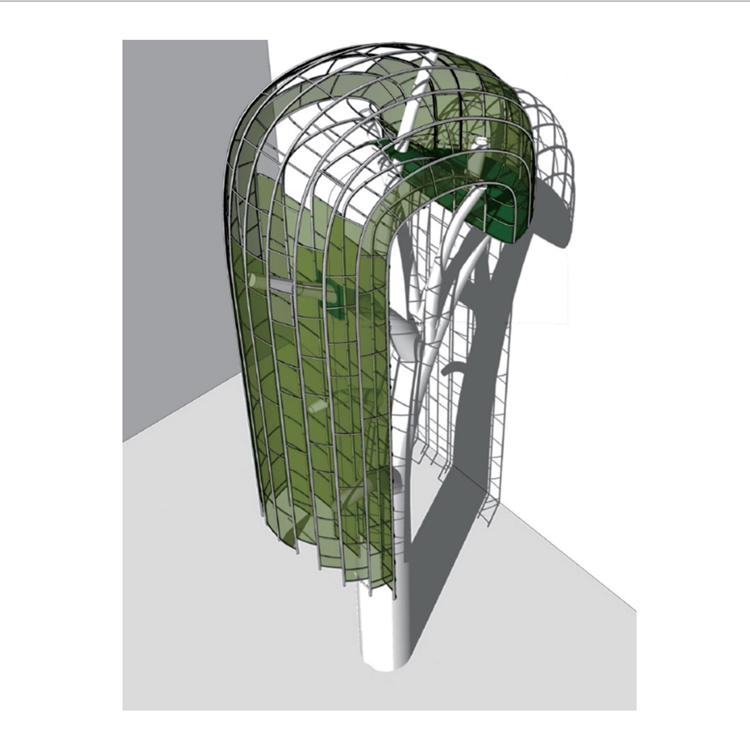# 锥形弯管

2019-12-10 14:44 孟村县浩德异型弯厂
21241*426*10   L=6m        245*440*10   L=6500mm

273*450*10   L=5.8m       60*50*4   L=1400

273*461*10   L=6m        76*60*4    L=800

194*362*6   L=3.8m         89*73*5   L=700

200*300*12   L=5m          140*114*6   L=1000

219*377*10   L=5.8m        168*114*6   L=1400

273*325*12   L=5.8m        203*340*8   L=3800

219*325*10   L=5.6m